# Literals

• reference
+
Literal values include strings, numbers, TRUE, FALSE, NULL, and MISSING.

SQL++ supports the same literals as JSON, as defined by json.org, with these exceptions:

• In SQL++, "true", "false," and "null" are case-insensitive to be consistent with other SQL++ keywords. In standard JSON, "true", "false," and "null" are case-sensitive.

• "missing" is added as a literal expression, although it is not returned in final results. Missing is omitted from objects, and is converted to null in result arrays.

• In SQL++ single and double quotation marks can be used for strings. JSON supports only double quotation marks.

Wherever a value is expected, either of two special values may appear: NULL (denoting an out-of-band value that is not comparable to any other value), and MISSING (denoting the absence of a value). Every value also has a "truth" value; these truth value conversions are explained in Boolean Logic.

## Booleans

``boolean ::= 'TRUE' | 'FALSE'``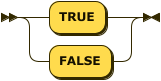Boolean propositions evaluate to TRUE and FALSE. These values are case-insensitive.

## Numbers

``number ::= '-'? integer fraction? exponent?````integer ::= [0-9] | [1-9] [0-9]+````fraction ::= '.' [0-9]+``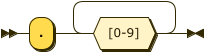``exponent ::= ('e' | 'E') ('-' | '+')? [0-9]+``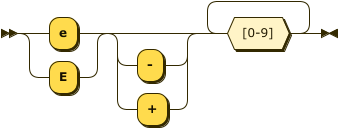Numbers can be either signed or unsigned integers with an optional fractional component and an optional exponent. If the integer component has more than one digit, the number should not start with a leading zero.

## Strings

``string ::= '"' char* '"' | "'" char* "'"``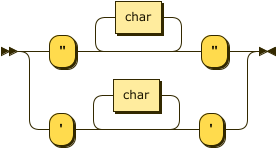``````char ::= unicode-character |
'\' ( '\' | '"' | "'" | 'b' | 'f' | 'n' | 'r' | 't' | 'u' hex hex hex hex )``````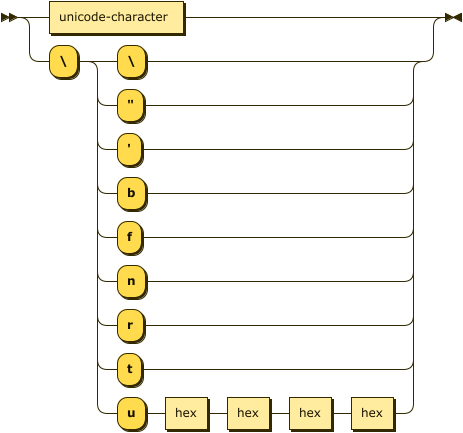``hex ::= [0-9a-fA-F]``Strings can be either Unicode characters or escaped characters.

## NULL

``null ::= 'NULL'``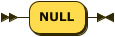The literal NULL represents an empty value. This value is case-insensitive.

## MISSING

``missing ::= 'MISSING'``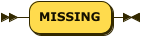The MISSING literal is specific to SQL++ and represents a missing name-value pair in a document. This value is case-insensitive.

Output